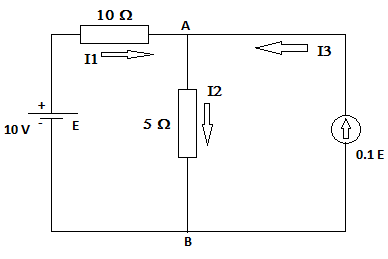# Find I_1, I_2, I_3 and V_{AB}.

## Question:

Find {eq}I_1, I_2, I_3 {/eq} and {eq}V_{AB} {/eq}.## Ohm's Law

Ohm's law states that current flowing across a wire is directly proportional to the voltage applied across the wire provided the Physical conditions like temperature, pressure, etc remains constant. Mathematically

{eq}\begin{align} V = IR \end{align} {/eq}

Where V is the voltage applied, {eq}I {/eq} is the current in the wire, R is called the resistance of the wire and depends upon the dimensions and nature of the material of the wire.

Data Given

• EMF of the battery {eq}E = 10.0 \ \rm V {/eq}

The voltage drop across the end AB as both the component are in parallel so the voltage drop across both the wires should be same

{eq}\begin{align} V_{AB} = 0.10 \ \rm E \\ V_{AB}= 0.10 \ \rm 10 \ \rm V \\ \color{blue}{\boxed{ \ V_{AB}= 1.0 \ \rm V \ }} \end{align} {/eq}

Now current across the 5 Ohm resistor as the voltage across this resistaor is {eq}V_{AB} {/eq} so using Ohm's law

{eq}\begin{align} I_2 = \frac{V_{AB}}{5 \ \rm \Omega} \\ I_2 = \frac{1.0 \ \rm V \ }{5 \ \rm \Omega } \\ \color{blue}{\boxed{ \ I_2 = 0.2 \ \rm A \ }} \end{align} {/eq}

Now current across the 10 ohm resistor

{eq}\begin{align} I_1 = \frac{E-V_{AB}}{10 \ \rm \Omega} \\ I_1 = \frac{10 \ \rm V - 1.0 \ \rm V \ }{10 \ \rm \Omega } \\ \color{blue}{\boxed{ \ I_1 = 0.99 \ \rm A \ }} \end{align} {/eq}

Finally using conservation of charge at point A

{eq}\begin{align} I_1 =I_2 + I_3 \\ I_3 = I_1 - I_2 \\ I_3 = 0.99 \ \rm A - 0.20 \ \rm A \\ \color{blue}{\boxed{ \ I_3 = 0.79 \ \rm A \ }} \end{align} {/eq}